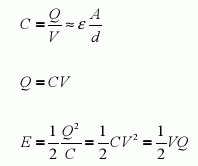Capacitors are electrical devices that are used to store electrical energy. A typical capacitor consists of two fixed conducting plates (electrodes) which face each other and are separated by a distance (d) An insulating material (such as air) fills the gap between the two plates. This insulating material is called the dielectric.
When a potential difference or voltage (V, volts) is applied across the two plates, an amount of opposite and equal charge (Q, coulombs) is stored in each electrode. Capacitance (F, farads) is the charge per unit volt that a capacitor can store, Because a farad is an extremely large unit, capacitances are measured normally in micro farads ( F), nano farads (nF), or pico farads (pF).
Because a capacitor’s plates are fixed, an electric field will develop. As a consequence of this, a voltage develops across the plates. As more charges accumulate, the voltage increases. The voltage of the applied potential is the maximum voltage that can appear across the capacitor. The amount of charge that can be stored in the plates is a function of the plate areas (A, in meters), the permittivity of the dielectric ( , in farads per meter), and the separation of the plates (d). The energy stored in the capacitor (E, joules), due to the accumulation of charges, is a measure of the amount of work needed to develop the voltage across the plates.